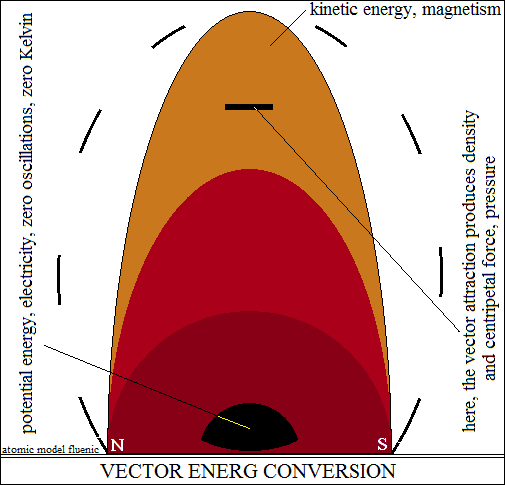Existence is vector energy.
The human species and unconscious development.

What does the human species represent for the planet and what does the planet
represent for the human species, given that the human species does not know
that the planet is the biological structure from which it was born? Multiply
yourselves! and we multiplied... With the current definition of energy, it is
very difficult to accept the internal structure of stars, with an electric core at
zero Kelvin temperature. The definition is a generator of scientific formalism
and dogmas, of falsehoods. Therefore, the definition produced the electron,
a lie bigger than the universe! In this article I describe the phenomena that produce
the internal structure of stars. The essence of vector energy is the unknowable
vector properties, perceived only as effects, through the organs of sense and
reason through measuring instruments. The properties of vector energy are
attractive forces and repulsive forces, which form pairs of orthogonally closed
circuits, one around the other. I have to admit, the vector being the symbol
of a phenomenon, was seen in my subconscious as a scalar quantity, which
in singular closed orthogonal circuits, explained by trigonometry the centripetal
force. Deeper thinking changed the shape of this interpretation, showing the
phenomena in a much more interesting form. Beyond the limit of sensory
perception, the effects of vector forces appear interdependent,
interacting as a whole, called vector energy. The force of attraction
is a variable quantity that forms density spectra.
The force of attraction, the queen of vector properties.
It is found that the attraction forces simultaneously generate orthogonally
closed vector circuits. It is deduced that the attraction forces between the
vector polarities of opposite sign in each circuit transform the energy of the
length of the circuit into density and implicitly into centripetal force, up to a
common central point. The phenomenon also explains the increase in the
centripetal force, the density of the vector space, inversely proportional
to the radius. Centripetal force is called gravity by Newton, and Einstein calls
it curved space, in reality it is the density spectrum of the oriented vector space.
The common central point, with the surrounding vectorial space polarized\
by the internal structure, is the nucleus of Rutherford's experiment.
experiment. Microscopically, the circuits contract and dilate mutually and
alternately, oscillating with their own resonant frequencies, around a balance.
At the astronomical level, orthogonally closed vector circuits cannot oscillate.
The repulsive forces of one (magnetic) circuit propagate orthogonally around
the other (electrical) circuit, forming a stable bipolar astronomical structure.
In this structure, the kinetic energy of the magnetic circuit is continuously
transformed into the potential energy of the electric circuit and vice versa.
The apparent centripetal force is derived from the vector property of attraction.
<< In my mind, the orthogonal circuits of vector, electric and magnetic energy,
represented by distinct lines, do not show reality. The huge potential energy
density in the center of the structure expands kinetically through the magnetic
poles, in the form of open polarities, with a potential similar to the Van de Graaff
generator. The polar potential of the open circuits curvilinearly polarizes the
vector space, at astronomical distances, the open circuits being homogeneously
oriented vector space. The circuits aligned in series the polarities of the vector
space with the forces of attraction, and the curvilinear, with the forces of
repulsion, the circuits having the same sense. Open circuits propagate like radio
waves, but without oscillations. In the equatorial plane, the polarizations of the
homogeneously oriented vector space close the circuit, delimited lentiformly.
In the lentiform space, the propagation of the forces of attraction continues,
generating an increase in the density of closed circuits, inversely proportional
to the radius of curvature, forming the density spectrum, with the maximum
limit in the electric circuit, zero oscillations, zero Kelvin. Obviously, interactions
occur at the speed of light. The intrusions of microscopic structures (of atoms)
into the lentiform space, suffer the interactions of the density, until suppression.>>
I briefly described the vector energy interactions that
form the internal structures of astronomical bodies.<
>